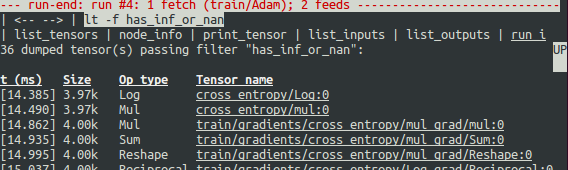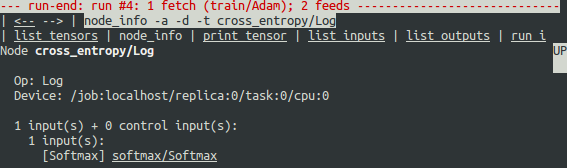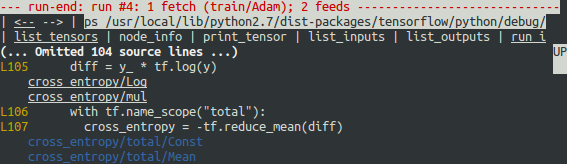## TensorFlow 学习 -- TensorFlow 调试程序（2）

695 0

### 快来加入 TensorFlowers 大家庭！

x

tfdbg CLI 的其他功能

• 要浏览之前的 tfdbg 命令，请输入几个字符，然后按向上或向下箭头键。tfdbg 会向您显示以这些字符开头的命令的历史记录。
• 要浏览屏幕输出的历史记录，请执行下列任一操作：
• 使用 prev 和 next 命令。
• 点击屏幕左上角附近带下划线的 <-- 和 --> 链接。
• 命令（和一些命令参数）的 Tab 补齐功能。
• 要将屏幕输出重定向到文件（而不是屏幕），请使用 bash 样式重定向结束命令。例如，以下命令会将 pt 命令的输出重定向到 /tmp/xent_value_slices.txt 文件：

tfdbg> pt cross_entropy/Log:0[:, 0:10] > /tmp/xent_value_slices.txt

tfdbg> run -t 10

tfdbg> run -f has_inf_or_nan

def my_filter_callable(datum, tensor):
# A filter that detects zero-valued scalars.
return len(tensor.shape) == 0 and tensor == 0.0

tfdbg> run -f my_filtertfdbg> pt cross_entropy/Log:0

tfdbg> /inf

tfdbg> /(inf|nan)

tfdbg> pt -s cross_entropy/Log:0

tfdbg> ni cross_entropy/Logtfdbg> pt Softmax:0

tfdbg> /0\.000

tfdbg> ni -t cross_entropy/Log

diff = y_ * tf.log(y)
tfdbg 有一个可以轻松将张量和指令追溯到 Python 源文件中的行的功能。它可以用行创建的指令或张量注解 Python 文件的行。要使用此功能，只需点击 ni -t <op_name> 命令的堆栈追踪输出中带下划线的行编号，或者使用 ps（或 print_source）命令，例如：ps /path/to/source.py。例如，以下屏幕截图显示了 ps 命令的输出。diff = -(y_ * tf.log(y))

diff = tf.losses.sparse_softmax_cross_entropy(labels=y_, logits=logits)

python -m tensorflow.python.debug.examples.debug_mnist --debug

run -f has_inf_or_nan`

# First, let your BUILD target depend on "//tensorflow/python/debug:debug_py"
# (You don't need to worry about the BUILD dependency if you are using a pip
#  install of open-source TensorFlow.)
from tensorflow.python import debug as tf_debug

# Create a LocalCLIDebugHook and use it as a monitor when calling fit().
hooks = [tf_debug.LocalCLIDebugHook()]

classifier.fit(x=training_set.data,
y=training_set.target,
steps=1000,
monitors=hooks)

accuracy_score = classifier.evaluate(x=test_set.data,
y=test_set.target,
hooks=hooks)["accuracy"]
debug_tflearn_iris.py（基于 tf-learn 的鸢尾花教程）包含如何搭配使用 tfdbg 和 Estimator 的完整示例。要运行此示例，请执行以下操作：

python -m tensorflow.python.debug.examples.debug_tflearn_iris --debug

tf.contrib.learn 中，Experiment 是一个比 Estimator 更高级别的构造。它提供了用于训练和评估模型的单个界面。为了调试对 Experiment 对象的 train() 和 evaluate() 调用，在调用该对象的构造函数时，您可以分别使用关键字参数 train_monitors 和 eval_hooks。例如：

# First, let your BUILD target depend on "//tensorflow/python/debug:debug_py"
# (You don't need to worry about the BUILD dependency if you are using a pip
#  install of open-source TensorFlow.)
from tensorflow.python import debug as tf_debug

hooks = [tf_debug.LocalCLIDebugHook()]

ex = experiment.Experiment(classifier,
train_input_fn=iris_input_fn,
eval_input_fn=iris_input_fn,
train_steps=FLAGS.train_steps,
eval_delay_secs=0,
eval_steps=1,
train_monitors=hooks,
eval_hooks=hooks)

ex.train()
accuracy_score = ex.evaluate()["accuracy"]

python -m tensorflow.python.debug.examples.debug_tflearn_iris \
--use_experiment --debug
LocalCLIDebugHook 还允许您配置 watch_fn，后者可用于灵活指定在不同的 Session.run() 调用期间要查看哪些 Tensor，这些调用作为 fetches 和 feed_dict 以及其他状态的函数。如需了解详情，请参阅此 API 文档

import tensorflow as tf
from keras import backend as keras_backend
from tensorflow.python import debug as tf_debug

keras_backend.set_session(tf_debug.LocalCLIDebugWrapperSession(tf.Session()))

# Define your keras model, called "model".
model.fit(...)  # This will break into the TFDBG CLI.本版积分规则 回帖后跳转到最后一页## 新帖排行